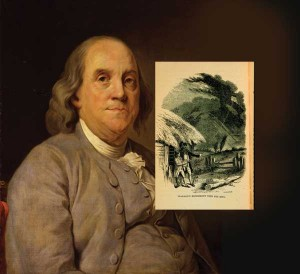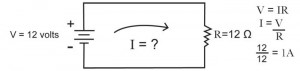# An Introduction to Electrical FundamentalsBy strict definition a material can be classified as either a conductor, an insulator, or a semiconductor based on its electrical properties. Before getting into the definition of each of these, a brief introduction to atomic theory is in order.

The atom is the smallest individual structure that makes up any chemical element while retaining properties of the element. The atom has a nucleus that contains protons and neutrons. Electrons orbit the nucleus at various distances and with varying amounts of energy. Protons are positively charged particles, electrons are negatively charged particles, and neutrons are uncharged particles. These electrons known asvalence electronsare what we are primarily interested in from an electrical perspective. The valence shell is the outermost orbiting shell around the nucleus of the atom. It is important to note that electrons in the valence shell have higher energy than those orbiting closest to the nucleus. The distance away from the nucleus also allows these valence electrons to escape easier. The electrons that are given up from the valence shell become known asfree electronsand contribute to current flow. Figure 1 shows the atomic structure of Hydrogen.

ConductorFigure 1. Atomic Structure of Hydrogen

A conductor has the property of conducting electrical current very easily. Examples of conductors are gold, silver, copper, and aluminum. Gold and silver are not used as conductors in electrical construction because they are extremely cost prohibitive. Copper and aluminum are the traditional conductors used in electrical construction. These chemical elements are characterized by atoms that contain electrons in the valence shell that are not tightly bound and can easily contribute to current flow. Most conductors are metals and have high conductivities based on their resistivities.

Insulator

An insulator has the property of not conducting electrical current very easily. Examples of insulators are glass, porcelain, rubber, and mica. Note how these products are not individual chemical elements but rather chemical compounds made up of different chemical elements. These chemical compounds are characterized by atoms that contain electrons in the valence shell that are tightly bound and do not easily contribute to current flow. Most insulators are non-metals and have low conductivities based on their resistivities.

Semiconductor

A semiconductor has the property of behaving like something in-between an insulator and conductor. Examples of semiconductors are silicon and germanium. Silicon and germanium in elemental form are poor insulators and poor conductors. But when combined with other chemical compounds these devices can become insulators, conductors, or variations of both. Through a process known as doping, impurities are added to elemental silicon or germanium allowing the electrical properties of these materials to be changed.

So we have now learned that conductors can conduct current very easily, and insulators cannot conduct current very easily. What exactly is current and how does it flow through a conductor? To understand how current flows through a conductor we have to discuss four important terms: voltage, current, resistance, and resistivity.

VoltageFigure 2. Example of Ohm’s Law

The technical definition of voltage is the unit of potential difference between two points when 1 joule (unit of energy) of work is expended to move a test charge of 1 coulomb (unit of electric charge) from point A to point B. The unit of voltage is the volt and the meter used to measure voltage is the voltmeter. The voltmeter is placed in parallel with the device under test. Depending on whether the voltage representation is an AC or DC waveform, terms you will encounter areAverage,RMS(root mean square), andP-P(peak to peak).

The technical definition of current is the movement of a test charge of 1 coulomb past a given point in 1 second. The unit of current is the ampere and the meter used to measure current is the ammeter. The ammeter is placed in series with the device under test.

The definition of resistance is the opposition to current flow that is measured when electric current flows through a conductor. The unit of resistance is the ohm and the meter used to measure resistance is the ohmmeter. A very useful equation known as Ohm’s Law is helpful in solving for the derived unit of resistance. See figure 2 for an example of Ohm’s Law.

Sometimes a mechanical analogy helps to keep these terms in perspective. Consider a garden hose that supplies the flow of water to a water fountain. The voltage would be the pressure of the water in the hose, the current would be the flow of water through the hose, and the resistance would be the friction the hose presents to the flow of water. The water fountain would be the load because it is performing some useful work.

ResistivityFigure 3. Ohm’s Law

The last term to define is resistivity. Resistivity is the resistance offered by a material to the flow of current designated by a specific length and specific cross-sectional area. The unit of resistivity is the circular mil-ohm/ft. One circular mil is the area of a circle having a diameter of 1 mil. One mil is 1/1000thof an inch. Copper is a conductor and therefore has a low resistivity and high conductivity. Rubber is an insulator and therefore has a high resistivity and low conductivity.

An example will help to clarify these points. Let’s take a #16 THHN copper conductor with a length of 100 ft. First recognize that the copper wire is a conductor and has a very low resistivity which means it will pass current very easily. Next recognize the THHN (thermoplastic insulation, high heat resistant, dry location only, nylon jacket) insulation is an insulator and has a very high resistivity which means it will not pass current very easily. From Table 8 Conductor Properties in Chapter 9 of theNEC,we find the diameter of #16 THHN to be 0.051 in. The resistivity of copper is 10.371 cmil-ohm/ft. Let’s calculate the resistance of 100 ft of this conductor.

L = 100 ft
Ρcu = 10.371 cmil − Ω ⁄ ft
D = 0.051 in = 51 mils
A = 2601 cmil
R (100 ft) = (ρ* (L)/A
= (10.371) * (100) / 2601

= 0.398 Ω

Voltage, current, resistance, and resistivity are important electrical units. Conductors and insulators are very common materials used every day in electrical construction. These core definitions will allow for further discussion of devices like resistors, capacitors, and inductors and how they perform in a variety of circuits and under a variety of conditions.

Stephen J. Vidal, P. E. is president of Joseph J. Vidal & Sons, Inc. in Throop, Pennsylvania. He has a B.S.E.E. with a minor in physics from Wilkes College in Wilkes Barre, Pennsylvania and a MBA from City University in Seattle, Washington. He is a registered professional engineer with the Commonwealth of Pennsylvania and holds a master contractor’s license in Wilkes Barre. He is also a general class amateur radio operator KA3KEM.